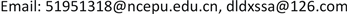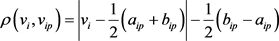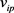﻿ 基于可拓模型的地方政府财政风险预警研究 A Study of Local Government Financial Risk Warning Based on Extenics Model

Service Science and Management
Vol. 07  No. 06 ( 2018 ), Article ID: 27535 , 8 pages
10.12677/SSEM.2018.76024

A Study of Local Government Financial Risk Warning Based on Extenics Model

—Taking the Financial Data of Beijing-Tianjin-Hebei as an Example

Shengan Shi1, Baokun Tian2, Yuqi Wang1

1Department of Law and Politics, North China Electric Power University, Baoding Hebei

2Department of Continuing Education, Hebei Science and Technology Engineering School, Baoding HebeiReceived: Oct. 26th, 2018; accepted: Nov. 7th, 2018; published: Nov. 14th, 2018ABSTRACT

With the gradual deepening of the coordinated development of Beijing-Tianjin-Hebei in recent years, the government cooperation in this region has been constantly strengthened. However, due to the different economic development and fiscal revenue and expenditure arrangements, there are different levels of financial risks between governments. It not only becomes a constraint factor for local governments to perform government functions, but also hinders the development process of Beijing-Tianjin-Hebei integration. Therefore, studying local financial risks, establishing an early warning mechanism to prevent and defuse financial risks in the Beijing-Tianjin-Hebei region can enable the government to take effective measures in the initial stage of possible fiscal risks to avoid further financial crises. By selecting dynamic and static financial risk indexes and using extenics model, this paper analyzes the data of the Beijing-Tianjin-Hebei region and puts forward corresponding countermeasures according to the results.

Keywords:Beijing-Tianjin-Hebei, Local Finance, Risk Warning, Extenics Model

—以京津冀三地财政数据为例

1华北电力大学法政系，河北 保定

2河北省科技工程学校，河北 保定Copyright © 2018 by authors and Hans Publishers Inc.

This work is licensed under the Creative Commons Attribution International License (CC BY).

http://creativecommons.org/licenses/by/4.0/1. 问题的提出

2. 模型的构建

(一) 财政风险预警指标的选取Table 1. Local government fiscal risk early warning index system

(二) 物元可拓模型的构建

1) 确定经典域

${R}_{0}=\left[\begin{array}{ccccc}N& {N}_{1}& {N}_{2}& \cdots & {N}_{m}\\ {c}_{1}& \left[{a}_{11},{b}_{11}\right]& \left[{a}_{12},{b}_{12}\right]& \cdots & \left[{a}_{1m},{b}_{1m}\right]\\ {c}_{2}& \left[{a}_{21},{b}_{21}\right]& \left[{a}_{22},{b}_{22}\right]& \cdots & \left[{a}_{2m},{b}_{2m}\right]\\ ⋮& ⋮& ⋮& \ddots & ⋮\\ {c}_{n}& \left[{a}_{n1},{b}_{n1}\right]& \left[{a}_{n2},{b}_{n2}\right]& \cdots & \left[{a}_{nm},{b}_{nm}\right]\end{array}\right]$

${C}_{i}$ 是第i个评价指标；

${v}_{ij}=\left[{a}_{ij},{b}_{ij}\right]$${N}_{j}$ 关于 ${c}_{i}$ 所规定的量值范围，即经典域。

2) 确定节域

${R}_{p}=\left(P,C,{V}_{p}\right)=\left[\begin{array}{ccc}P& {c}_{1}& \left[{a}_{1p},{b}_{1p}\right]\\ & {c}_{2}& \left[{a}_{2p},{b}_{2p}\right]\\ & ⋮& ⋮\\ & {c}_{n}& \left[{a}_{np},{b}_{np}\right]\end{array}\right]$是P关于 ${c}_{i}$ 所取的量值范围，即节域。

3) 确定待评价物元

${v}_{i}$ 是待评价事物对应于 ${c}_{i}$ 的数值。

4) 确定评价指标的权重

${W}_{i}\ge 0\left(i=1,2,\cdots ,n\right)$

$\sum _{i=1}^{n}{W}_{i}=1$

5) 确定关联度

$\rho \left({v}_{i},{v}_{ij}\right)=|{v}_{i}-\frac{1}{2}\left({a}_{ij}+{b}_{ij}\right)|-\frac{1}{2}\left({b}_{ij}-{a}_{ij}\right)$$\rho \left({v}_{i},{v}_{ip}\right)$ 是点 ${v}_{i}$ 与区间的距。

${K}_{j}\left({v}_{i}\right)=\left\{\begin{array}{ll}\frac{\rho \left({v}_{i},{v}_{ij}\right)}{\rho \left({v}_{i},{v}_{ip}\right)-\rho \left({v}_{i},{v}_{ij}\right)}\hfill & {v}_{i}\notin {v}_{ij}\hfill \\ \frac{-\rho \left({v}_{i},{v}_{ij}\right)}{|{v}_{ij}|}\hfill & {v}_{i}\in {v}_{ij}\hfill \end{array}$

${K}_{j}\left({v}_{i}\right)$ 是关联函数，待评价事物的指标 ${c}_{i}$ 关于类别j的归属度；

$|{v}_{ij}|$ 是区间 $\left[{a}_{ij},{b}_{ij}\right]$ 的长度，即${K}_{j}\left(p\right)=\sum _{i=1}^{n}{W}_{i}{K}_{j}\left(vi\right)$

6) 确定待评事物的类别$\left(j=1,2,\cdots ,m\right)$ ，则评定p属于类别j0

3. 实证分析

(一) 数据的选取

(二) 分析指标预警界限值的确定

(三) 结构指标权重的确定

(四) 结果分析Table 2. Fiscal data of the three provinces of Beijing-Tianjin-Hebei in 2011-2015Table 3. Financial data indicators early warning threshold intervalTable 4. Financial data analysis results of the three provinces of Beijing-Tianjin-Hebei

4. 政策建议

(一) 建立完善的财政风险预警系统

(二) 优化地方政府支出结构

(三) 转变政府职能，加强政府间合作

A Study of Local Government Financial Risk Warning Based on Extenics Model[J]. 服务科学和管理, 2018, 07(06): 197-204. https://doi.org/10.12677/SSEM.2018.76024

1. 1. 徐珊珊. 我国地方财政风险问题研究[D]: [硕士学位论文]. 合肥: 安徽财经大学, 2015.

2. 2. Polackova, H. (1998) Contingent Government Liabilities: A Hidden Risk for Fiscal Stability. Policy Research Working Paper.

3. 3. Easterly, W. and Yuravlivker, D. (2002) Evaluating Government NetWorth in Colombia and Republica Bolivariana de Venezuela. Government at Risk: Contingent Liabilities and Fiscal Risk, 181-202.

4. 4. Hemming, R. and Petrie, M. (2002) A Framework for Assessing Fiscal Vulnerability. Government at Risk: Contingent Liabilities and Fiscal Risk, 159.

5. 5. Hildreth, W.B. (2002) Debt and the Local Economy: Problems in Benchmarking Local Government Debt Affordability. Public Budgeting & Finance, 22, 99-113. https://doi.org/10.1111/1540-5850.00091

6. 6. 王亚芬, 梁云芳. 我国财政风险预警系统的建立与应用研究[J]. 财政研究, 2004(11): 25-27.

7. 7. 刘尚希. 财政风险: 从经济总量角度的分析[J]. 管理世界, 2005(7): 31-39.

8. 8. 洪源. 中国财政风险非参数预警系统构建与实证分析——基于风险因子和AHP法的研究[J]. 河北经贸大学学报, 2011(5): 44-51.

9. 9. 章志平. 中国地方政府债务风险灰色评估和预警[J]. 统计与决策, 2011(15): 135-138.

10. 10. 郭玉清. 逾期债务、风险状况与中国财政安全[J]. 经济研究, 2011(8): 38-50.

11. 11. 吴海蓉. 地方政府债务存在的原因和风险探索[D]: [硕士学位论文]. 上海: 华东理工大学, 2013.

12. 12. 吕奕星. 我国地方政府债务风险问题研究[D]: [硕士学位论文]. 郑州: 郑州大学, 2013.

13. 13. 刘谊, 刘星, 马千真, 等. 地方财政风险监控体系的建立及实证分析[J]. 中央财经大学学报, 2004(7): 1-5.

14. 14. 马恩涛. 我国财政风险预警系统研究[J]. 山东经济, 2007(4): 84-88.

15. 15. 蔡文. 可拓学概论[J]. 系统工程理论与实践, 1998(1): 76-84.

16. 16. 蔡文, 杨春燕, 何斌. 可拓逻辑初步[M]. 北京: 科学出版社, 2003: 8-25.

17. 17. 蔡文, 杨春燕, 林伟初. 可拓工程方法[M]. 北京: 科学出版社, 2000: 4-15.

18. 18. 蔡文. 物元模型及其应用[M]. 北京: 科学技术文献出版社, 1998: 267-275.